# Samacheer Kalvi Books: Tamilnadu State Board Text Books Solutions

## Samacheer Kalvi 9th Science Chapter 7 Heat Notes PDF Download: Tamil Nadu STD 9th Science Chapter 7 Heat NotesSamacheer Kalvi 9th Science Chapter 7 Heat Notes PDF Download: Tamil Nadu STD 9th Science Chapter 7 Heat Notes

We bring to you specially curated Samacheer Kalvi 9th Science Chapter 7 Heat Notes PDF which have been prepared by our subject experts after carefully following the trend of the exam in the last few years. The notes will not only serve for revision purposes, but also will have several cuts and easy methods to go about a difficult problem.

 Board Tamilnadu Board Study Material Notes Class Samacheer Kalvi 9th Science Subject 9th Science Chapter Chapter 7 Heat Format PDF Provider Samacheer Kalvi Books

## How to Download Samacheer Kalvi 9th Science Chapter 7 Heat Notes PDFs?

2. Click on the Samacheer Kalvi 9th Science Notes PDF.
3. Look for your preferred subject.
4. Now download the Samacheer Kalvi 9th Science Chapter 7 Heat notes PDF.

## Download Samacheer Kalvi 9th Science Chapter 7 Heat Chapterwise Notes PDF

### Samacheer Kalvi 9th Science Heat Textbook Exercises

Heat Lesson For 9th Class Question 1.
Calorie is the unit of
(a) heat
(b) work
(c) temperature
(d) food
(a) heat

9th Class Physics Heat Lesson Question 2.
SI unit of temperature is
(a) fahrenheit
(b) joule
(c) Celsius
(d) kelvin
(d) kelvin

Heat Samacheer Kalvi Question 3.
Two cylindrical rods of same length have the area of cross-section in the ratio 2:1. If both the rods are made up of same material, which of them conduct heat faster?
(a) Both rods
(b) Rod-2
(c) Rod-1
(d) None of them
(c) Rod-1

Heat Lesson In Physics For Class 9 Question 4.
In which mode of transfer of heat, molecules pass on heat energy to neighbouring molecules without actually moving from their positions?
(b) Conduction
(c) Convection
(d) Both B and C
(b) Conduction

9th Class Physical Science Heat Lesson Question 5.
A device in which the loss of heat due to conduction, convection and radiation is minimized is
(a) Solar cell
(b) Solar cooker
(c) Thermometer

1.  (a) Heat
2.  (d) kelvin
3.  (c) Rod-1
4.  (b) Conduction

II. Fill in the blanks.

1. The fastest mode of heat transfer is ……………….
2. During day time, air blows from ………… to …………….
3. Liquids and gases are generally …………….. conductors of heat.
4. The fixed temperature at which matter changes state from solid to liquid is called ………………

2. land, sea
4. melting point

III. Assertion and Reason type questions.

Mark the correct choice as:
(a) If both assertion and reason are true and reason is the correct explanation of assertion.
(b) If both assertion and reason are true but reason is not the correct explanation of assertion.
(c) If assertion is true but reason is false.
(d) If assertion is false but reason is true.

Chapter 7 Heat Question 1.
Assertion(A): Food can be cooked faster in copper bottom vessels.
Reason (R): Copper is the best conductor of heat.
(a) If both assertion and reason are true and reason is the correct explanation of assertion.

Heat 9th Class Question 2.
Assertion(A): Maximum sunlight reaches earth’s surface during the afternoon time.
Reason(R): Heat from the sun reaches earth’s surface by radiation.
(b) If both assertion and reason are true but reason is not the correct explanation of assertion.

7 Heat Question 3.
Assertion(A): When water is heated up to 100°C, there is no raise in temperature until all water gets converted into water vapour.

Reason(R): Boiling point of water is 10°C.
(c) If assertion is true but reason is false.

Lesson 7 Science Class 9 Question 1.
Define conduction.
The process of transfer of heat in solids from a region of higher temperature to a region of lower temperature without the actual movement of molecules is called conduction.

Samacheer Kalvi 9th Science Question 2.
Ice is kept in a double-walled container. Why?
Ice is kept in a double-walled container so as to prevent melting of ice from the heat absorbed present in the immediate surroundings. The vacuum present in between the two walls prevents the transfer of heat from the first to the second wall and hence the ice remains in the solid form for a longer time period.

Define Sublimation Class 9 Samacheer Kalvi Question 3.
How does the water kept in an earthen pot remain cool?
An earthen pot consists of small pores from which the water inside the pot constantly seeps out and gets evaporated due to the presence of high temperature around it. The evaporation process requires heat which is acquired from the surface of the pot, hence making the water and the pot cooler.

Samacheer Kalvi 9th Science Practical Question 4.

 S.No. Convection Radiation 1. Flow of heat through a fluid from places of higher temperature to places of lower temperature by movement of the fluid itself. Flow of heat from one place to another by means of electromagnetic waves. 2. Convection needs matter to be present. Radiation can occur even in vacuum. 3. Convection seen in daily life:   Hot air balloons, breeze, wind, chimney. Radiation in daily life:   White or light coloured cloths, highly polished surface of airplane, helps to reflect most of the heat radiation from the sun.

Samacheer Kalvi 9th Science Solutions Question 5.
Why do people prefer wearing white clothes during summer?
People prefer white or light coloured clothes during summer as they are good reflectors of heat and hence, they keep us cool.

Question 6.
What is specific heat capacity?

• The specific heat capacity of a substance is defined as the amount of heat required to raise the temperature of 1 kg of the substance by 1°C or 1 K.
• Q = mC∆T, where Q is the quantity of heat required to raise the temperature and m is the mass of the body and AT is the change in temperature of the body.
• The SI unit of specific heat capacity is Jkg– 1 K– 1. The most commonly used units of specific heat capacity are J/kg°C and J/g°C.

Question 7.
Define thermal capacity.

• Heat capacity or thermal capacity is defined as the amount of heat energy required to raise the temperature of a body by 1°C. It is denoted by ‘C’.
• C = Q/t, where C’ is the heat capacity, ‘Q’ is the quantity of heat required and ‘f’ is rise in temperature.
• SI unit of heat capacity is J/K. It is also expressed in cal/°C, kcal/°C or J/°C.

Question 8.
Define specific latent heat capacity.

• Specific latent heat is the amount of heat energy absorbed or liberated by unit mass of a substance during change of state without causing any change in temperature.
• Specific latent heat is given as L = Q/m, where ‘Q’ is the amount of heat energy absorbed or liberated and ‘m’ is mass of a substance during its change of phase at a constant temperature.
• The SI unit of specific latent heat is J/kg.

Question 1.
Explain convection in daily life.
Convection in daily life:
(i) Hot air balloons: Air molecules at the bottom of the balloon get heated by a heat source and rise. As the warm air rises, cold air is pushed downward and it is also heated. When the hot air is trapped inside the balloon, it rises.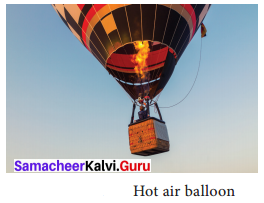(ii) Breeze: During day time, the air in contact with the land becomes hot and rises. Now the cool air over the surface of the sea replaces it. It is called sea breeze. During night time, air above the sea is warmer. As the warmer air over the surface of the sea rises, cooler air above the land moves towards the sea.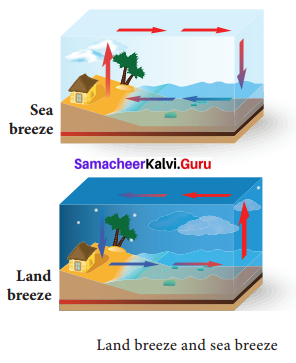(iii) Winds: Air flows from area of high pressure to area of low pressure. The warm air molecules over hot surface rise and create low pressure. So, cooler air with high pressure flows towards low pressure area. This causes wind flow.
(iv) Chimneys: Tall chimneys are kept in kitchen and industrial furnaces. As the hot gases and smoke are lighter, they rise up in the atmosphere.

Question 2.
What are the changes of state in water? Explain.
Any matter around us can be in three forms: solid, liquid and gas, called as states of matter. Depending upon the temperature, pressure and transfer of heat, matter is converted from one state to another and is known as change of state in matter. There are different such processes in the change of state in matter.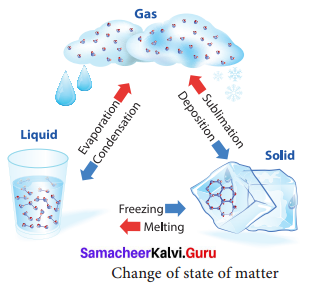For example;

• Water molecules are in liquid state at normal temperature. When water is heated to 100°C, it becomes steam or vapour which is a gaseous state of matter. The process by which a
liquid is converted to vapour by absorbing heat is called boiling or vaporization.
• The temperature at which a liquid changes its state to gas is called boiling point.
• On reducing the temperature of the steam it becomes water again. The process by which a vapour is converted to liquid by releasing heat is called condensation. On reducing the temperature of water further to 0°C, it becomes ice which is a solid state of water.
• The process by which a liquid is converted to solid by releasing heat is called freezing. The
temperature by which a liquid changes its state to solid is called freezing point. Ice on
heating, becomes water again by absorbing heat, a process known as melting.
• Dry ice changes directly to gaseous state without becoming liquid. This process is called
sublimation.

Thus, water changes its state when there is a change in temperature.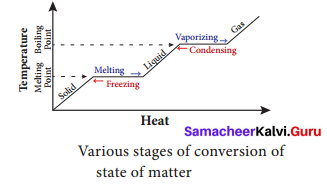Question 3.
How can you experimentally prove that water is a bad conductor of heat? How is it possible to heat water easily while cooking.
Experiment to prove that water is a bad conductor of heat:
Take a glass tube and drop an ice cube wrapped in wire gauze in it.
Now fill 3/4th of this tube with water and place it above the burner as shown in the figure.
You can observe that the water boils at the edge and the ice present in the bottom of the tube has not melted indicating that heat has not reached the bottom where the ice cube is present. This proves that water is a bad conductor of heat.
It is easy to heat water easily or quickly while cooking. This is because, while cooking the vessel or pan is usually covered with a lid.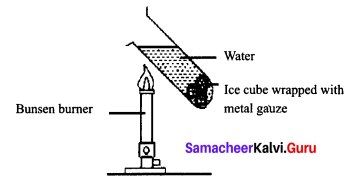1. Radiation from the hot water is reflected back into the pan rather than being emitted
2. Free convection is effectively eliminated, and
3. Evaporative cooling’ is also eliminated.
This in turn allows the water to be heated more easily.

VI. Problems:

Question 1.
What is the heat in joules required to raise the temperature of 25 grams of water from 0°C to 100°C? What is the heat in Calories? (Specific heat of water = 4.18 J/g°C).
Solution:
Given m = 25 g, ∆T = (100 – 0) = 100°C
Or in terms of Kelvin (373.15 – 273.15) = 100K,
C = 4.18J/g°C
Heat energy required, Q = m × C × ∆T = 25 × 4.18 × 100 = 10450 J

Question 2.
What could be the final temperature of a mixture of 100 g of water at 90°C and 600g of water at 20°C.
Solution:
To find final temperature: ∆Q = mc
lOOg of water originally at 90°C will loose an amount of heat,
∆Q = mc ∆T
∆Q = 100 × c × (90 – T)
The same amount of heat will be absorbed by 600g of water originally at 20°C to raise its temperature to T.
∆Q = 600 × c × (T – 30)
600C (T – 20°) = 100C (90° – T)
6T – 120° = 90° – T
6T + T = 120° + 90°
7T = 210° ⇒ T = 210/7
T = 30°C

Question 3.
How much heat energy is required to change 2 kg of ice at 0°C into water at 20°C? (Specific latent heat of fusion of water = 3,34,000J/kg, Specific heat capacity of water = 4200Jkg– 1 K– 1 ).
Solution:
Total heat = Heat required to convert 2Kg of ice into water at 0°C + Heat required to convert 2Kg of water at 0°C to 2Kg of water at 20°C
Heat = m (hfw) + mc∆T
Here, m(mass of ice) = 2Kg
hfw (specific latent heat of water) = 3,34,000J/Kg
C (specific heat capacity of water) = 4200JKg– 1K– 1
AT (Temperature difference) = 20°C
Therefore, Heat required = (2 × 334000) + (2 × 4200) (20 – 0)
= 668000 + 8400 (20)
= 668000+ 168000
Heat required = 8,36,000 J

Activity

Question 1.
Take a glass of water and put some ice cubes into it. Observe it for some time. What happens? The ice cubes melt and disappear. Why did it happen?
Solution:
It is because heat energy in the water is transferred to the ice.

Question 2.
Take metal rods of copper, aluminium, brass and iron. Fix a match stick to one end of each rod using a little melted wax. When the temperature of the far ends reach the melting point of wax, the matches drop off. It is observed that the match stick on the copper rod would fall first, showing copper as the best conductor followed by aluminum, brass and then iron.
You can do the activity by yourself.

Question 3.
Drop a few crystals of potassium permanganate down to the bottom of a beaker containing water. When the beaker is heated just below the crystals, by a small flame, purple streaks of water rise upwards and fan outwards.
You can do the activity by yourself.

Question 4.
Take some crushed ice cubes in a beaker and note down the temperature using thermometer. It will be 0°C. Now heat the ice i n the beaker. You can observe that ice is melting to form water. Record the temperature at regular intervals and it will remain at 0°C until whole ice is converted to liquid. Now heat the beaker again and record the temperature. You can notice that the temperature will rise up to 100°C and it will be at 100°C even after continuous heating until the whole mass of water in the beaker is vaporized.
You can do the activity by yourself.

### Samacheer Kalvi 9th Science Heat In Text Problems

Question 1.
Convert the following,

1.  25 °C to Kelvin
2. 200 K to °C

Solution:

1. Tk = T°C + 273.15
Tk = 25 + 273.15 = 298.15 K
2. T°C = Tk – 273.15
T°C = 200 – 273.15 = – 73.15 °C

Question 2.
Convert the following.

1. 35° C to Fahrenheit (°F)
2. 14 °F to °C

Solution:

1. T°F = T°C × 1.8 + 32
T°F = 25° C × 1.8 + 32 = 77 °F
2. T°C = (T°F – 32)/1.8
T°C = (14°F – 32)/1.8 = – 10 °C

Question 3.
Calculate the heat energy required to raise the temperature of 2 kg of water from 10°C to 50°C. Specific heat capacity of water is 4200 JKg– 1 K– 1.
Solution:
Given m = 2 Kg, ∆T = (50 – 10) = 40°C
In terms of Kelvin, ∆T = (323.15 – 283.15) = 40K,
C = 4200 J Kg– 1 K,sup>- 1
∴ Heat energy required, Q = m × C × ∆T = 2 × 4200 × 40 = 3,36,000 J

Question 4.
An iron ball requires 5000 J heat energy to raise its temperature by 20°C. Calculate the heat capacity of the iron ball.
Solution:
Given, Q = 5000 J, ∆T = 20°C or 20 K
Heat Capacity =  Heat energy required, Q Rise in temperature, ΔT=500020 = 250 JK– 1

Question 5.
How much heat energy is required to melt 5 kg of ice? (Specific latent heat of ice = 336 Jg– 1)
Solution:
Given, m = 5 Kg = 5000g, L = 336 Jg– 1
Heat energy required = m × L
= 5000 × 336
= 1680000J or 1.68 × 106J

Question 6.
How much boiling water at 100°C is needed to melt 2 kg of ice so that the mixture which is all water is at 0°C?
[Specific heat capacity of water = 4.2 JKg– 1 and specific latent heat of ice = 336 Jg– 1].
Solution:
Given, mass of ice = 2 kg = 2000 g.
Let m be the mass of boiling water required.
Heat lost = Heat gained.
m × c × ∆t = m × L
m × 4.2 × (100 – 0) = 2000 × 336
m = 2000×3364.2×100
= 1600 g or 1.6 kg.

### Samacheer Kalvi 9th Science Heat Additional Questions

Question 1.
Radiation is a method of heat transfer that does not require particles to carry the heat energy.

Question 2.
What is heat energy?
The sum of the kinetic and potential energy is called the internal heat energy of the molecules. This internal energy when flows out is called heat energy.

Question 3.
What are the three scales of temperature?
There are three scales of temperature.

1. Fahrenheit scale
3. Kelvin or Absolute scale.

Question 4.
What is absolute zero?
The temperature at which the pressure and volume of a gas theoretically reaches zero is called absolute zero.

Question 5.
What is the concept of temperature?
Temperature is the degree of hotness or coolness of a body. The hotter the body is higher is its temperature.

Question 6.
Differentiate between the three temperature scales.

 Fahrenheit Scale Celcius Scale Kelvin Scale 32°F and 212°F are the freezing and boiling points respectively. 0°C and 100°C are the freezing and boiling points respectively. OK represents absolute zreo. 373.15k and 273.15k are boiling and freezing points respectively. The interval has been divided into 180 parts. The interval has been divided into 100 parts. A one degree difference in kelvin is equal to one degree difference in celcius. The formula for converting a Fahrenheit scale to Celcius scale is – C = 5/9(F-32) The formula for converting a Celcius scale to Fahrenheit scale is   – F = 9/5C + 32 The formula for converting a Kelvin scale to Celcius scale is   -C = K-273.15 Also known as centigrade scale. Also known as absolute scale.

Question 1.
Write specific heat capacity of any five substances.
Specific heat capacity of a substance is defined as the amount of heat required to raise the temperature of 1 kg of the substance by 1°C or 1 K. The SI unit of specific heat capacity is Jkg– 1K– 1.

Specific Heat Capacity of some common substances

 S. No. Substance Specific Heat Capacity in JKg-1 KT1 1. Lead 130 2. Mercury 139 3. Brass 380 4. Zinc 391 5. Copper 399 6. Iron 483 7. Glass (flint) 504 8. Aluminium 882 9. Kerosene 2100 10. Ice 2100 11. Sea Water 3900 12. Water 4180

Question 2.
Differentiate between Conduction, Convection and Radiation.

 Conduction Convection Radiation Conduction takes place in solids Convection takes place in liquids and gases. All hot objects give away heat by radiation. Heat transfer takes place on contact between hot to cold molecule. The hot molecules goes away from the heat source to the coldest region to the top. Heat is carried as small packets of energy, which travel in straight line. Conduction does not occur in vacuum. Convection does not occur in vacuum. Radiation can occur in vacuum. Heat transfer can occur in any direction from hot to cold body. Heat transfer occurs along the path of convection current from heat source to the coldest region on the top. Heat transfer occurs when photons are met with an obstacle. Conduction is a relatively slow process. This process is faster than conduciton, but slower than radiation Radiation is fastest way of heat transfer.

Question 3.
What is latent heat of vapourisation. Explain with diagram.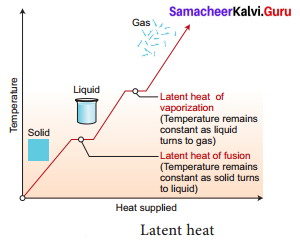Heat energy is absorbed by a solid during melting and an equal amount of heat energy is liberated by the liquid during freezing, without any temperature change. It is called latent heat of fusion. In the same manner, heat energy is absorbed by a liquid during vaporization and an equal amount of heat energy is liberated by the vapor during condensation, without any temperature changes. This is called latent heat of vaporization.

Question 4.
Give reasons why –

1. a steam burn is more damaging than a burn caused from boiling water at the same temperature.
2. an igloo is built as a winter shelter
3. gaps are left between railway tracks
4. chimneys are located high up in kitchens and factories
5. we wear woollen clothes in winter.

1. A steam bum is more damaging as when steam hits our skin it condenses to water and attains the temperature of our skin. There will be an energy release due to latent heat and fall in temperature. Whereas when boiling water fall on our skin, there is no transition in energy, only fall in temperature and heat transferred to the skin will be due to cooling.
2. igloo is built as a winter shelter due to snow’s insulating property. The inside of an igloo acts as an insulator and keeps it relatively warm.
3. During summer, heat causes expansion in tracks that are made of metal. If gaps a re not left between tracks, the track may bend due to expansion and accidents can occur.
4. As hot gases and smoke are lighter, they rise up. Therefore chimneys are placed high up in kitchens and factories, so that the hot air can rise up and go out into the atmosphere.
5. We wear woollen clothes in winter, as wool acts as an insulator. The air trapped between the woollen sweater and our clothes acts as an insulator and does not allow air from outside to enter, thereby keeping us warm.

## How to Prepare using Samacheer Kalvi 9th Science Chapter 7 Heat Notes PDF?

Students must prepare for the upcoming exams from Samacheer Kalvi 9th Science Chapter 7 Heat Notes PDF by following certain essential steps which are provided below.

• Use Samacheer Kalvi 9th Science Chapter 7 Heat notes by paying attention to facts and ideas.
• Pay attention to the important topics
• Refer TN Board books as well as the books recommended.
• Correctly follow the notes to reduce the number of questions being answered in the exam incorrectly
• Highlight and explain the concepts in details.

## Frequently Asked Questions on Samacheer Kalvi 9th Science Chapter 7 Heat Notes

#### How to use Samacheer Kalvi 9th Science Chapter 7 Heat Notes for preparation??

Read TN Board thoroughly, make separate notes for points you forget, formulae, reactions, diagrams. Highlight important points in the book itself and make use of the space provided in the margin to jot down other important points on the same topic from different sources.

#### How to make notes for Samacheer Kalvi 9th Science Chapter 7 Heat exam?

Read from hand-made notes prepared after understanding concepts, refrain from replicating from the textbook. Use highlighters for important points. Revise from these notes regularly and formulate your own tricks, shortcuts and mnemonics, mappings etc.
Share: